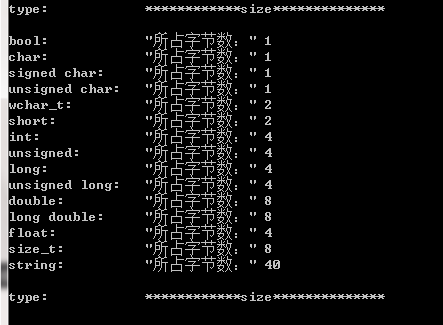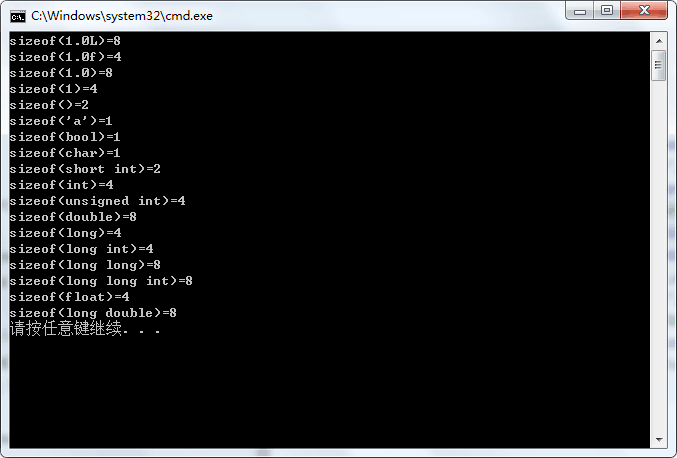• 2020-10-29 14:46:59

整型：byte(字节型，占1字节)、short(短整型，占2字节)、int(整型，占4字节)、long(长整型，占8字节）

	一般整型取值范围是分为有符号取值和无符号取值两种，有符号取值就把第一位取为符号位，无符号取值默认为大于等于0的整数。

例如：int 占4字节，一字节是8位，总共就是32位，它的有符号取值范围就是-2^31^  ~  2^31^-1  无符号取值为0  ~  2^32^-1


浮点型：float(单精度浮点数，占4字节)、double(双精度浮点数，占8字节)

​ 注：Java浮点数默认类型是double，float浮点数后缀为F(或f)

布尔型：boolean(布尔型)，只有true 和false两个取值

字符型：char(字符型，占2个字节)

​ 注：因字符类型表示Unicode字符，所以一个字符占2字节java
更多相关内容
• 综上，中文字符在编码中占用的字节数一般是2-4个字节。 int，longint，shortint的宽度都可能随编译器而异。但有几条铁定的原则（ANSI/ISO制订的）： sizeof(shortint)<=sizeof(int) sizeof(int)<=...

指针大小与系统编译器位数相同，16位编译器（2字节）、32位编译器（4字节）、64位编译器（8字节）

编码与中文：
Unicode/GBK： 中文2字节
UTF-8： 中文通常3字节，在拓展B区之后的是4字节
综上，中文字符在编码中占用的字节数一般是2-4个字节。

int，long int，short int的宽度都可能随编译器而异。但有几条铁定的原则（ANSI/ISO制订的）：

• sizeof(short int)<=sizeof(int)

• sizeof(int)<=sizeof(long int)

• short int至少应为16位（2字节）

• long int至少应为32位。

下面给出不同位数编译器下的基本数据类型所占的字节数：

16位编译器
char ：1个字节
char*(即指针变量): 2个字节
short int : 2个字节
int：  2个字节
unsigned int : 2个字节
float:  4个字节
double:   8个字节
long:   4个字节
long long:  8个字节
unsigned long:  4个字节

32位编译器
char ：1个字节
char*（即指针变量）: 4个字节（32位的寻址空间是2^32, 即32个bit，也就是4个字节。同理64位编译器）
short int : 2个字节
int：  4个字节
unsigned int : 4个字节
float:  4个字节
double:   8个字节
long:   4个字节
long long:  8个字节
unsigned long:  4个字节

64位编译器

char ：1个字节
char*(即指针变量): 8个字节
short int : 2个字节
int：  4个字节
unsigned int : 4个字节
float:  4个字节
double:   8个字节
long:   8个字节
long long:  8个字节

unsigned long:  8个字节

--------------------------------------------------------------------------------------------------------------------------------

Java中一共有8种基本数据类型，其中有4种整型，2种浮点类型，1种用于表示Unicode编码的字符单元的字符类型和1种用于表示真值的boolean类型。（一个字节等于8个bit）

1.整型
类型              存储需求     bit数    取值范围      备注
int                 4字节           4*8
short             2字节           2*8    －32768～32767
long              8字节           8*8
byte              1字节           1*8     －128～127

2.浮点型
类型              存储需求     bit数    取值范围      备注
float              4字节           4*8                  float类型的数值有一个后缀F(例如：3.14F)
double          8字节           8*8                       没有后缀F的浮点数值(如3.14)默认为double类型

3.char类型
类型              存储需求     bit数     取值范围      备注
char              2字节          2*8

4.boolean类型
类型              存储需求    bit数    取值范围      备注
boolean        1字节          1*8      false、true

补充：Java有一个能够表示任意精度的算书包，通常称为“大数值”(big number)。虽然被称为大数值，但它并不是一种Java类型，而是一个Java对象。

如果基本的整数和浮点数精度不能够满足需求，那么可以使用java.math包中的两个很有用的类：BigIntegerBigDecimal(Android SDK中也包含了java.math包以及这两个类)这两个类可以处理包含任意长度数字序列的数值。BigInteger类实现了任意精度的整数运算，BigDecimal实现了任意精度的浮点数运算。具体的用法可以参见Java API。

展开全文• void DataLength() { qDebug() << “type: \t\t” << “size**” << endl; qDebug() <... QString::fromLocal8Bit(“所占字节数：”) <... QString::fromLocal8Bit(“所占字节数：”) <
void DataLength()
{
qDebug() << "type: \t\t" << "************size**************" << endl;
qDebug() << "bool: \t\t" << QString::fromLocal8Bit("所占字节数：") << sizeof(bool);
qDebug() << "char: \t\t" << QString::fromLocal8Bit("所占字节数：") << sizeof(char);
qDebug()  << "signed char: \t" << QString::fromLocal8Bit("所占字节数：") << sizeof(signed char);
qDebug()  << "unsigned char: \t" << QString::fromLocal8Bit("所占字节数：") << sizeof(unsigned char);
qDebug()  << "wchar_t: \t" << QString::fromLocal8Bit("所占字节数：" )<< sizeof(wchar_t);
qDebug()  << "short: \t\t" << QString::fromLocal8Bit("所占字节数：") << sizeof(short);
qDebug()  << "int: \t\t" << QString::fromLocal8Bit("所占字节数：") << sizeof(int);
qDebug()  << "unsigned: \t" <<QString::fromLocal8Bit( "所占字节数：") << sizeof(unsigned);
qDebug()  << "long: \t\t" << QString::fromLocal8Bit("所占字节数：" )<< sizeof(long);
qDebug()  << "unsigned long: \t" <<QString::fromLocal8Bit( "所占字节数：" )<< sizeof(unsigned long);
qDebug()  << "double: \t" << QString::fromLocal8Bit("所占字节数：") << sizeof(double);
qDebug()  << "long double: \t" << QString::fromLocal8Bit("所占字节数：") << sizeof(long double);
qDebug()  << "float: \t\t" << QString::fromLocal8Bit("所占字节数：" )<< sizeof(float);
qDebug()  << "size_t: \t" << QString::fromLocal8Bit("所占字节数：") << sizeof(size_t);
qDebug()  << "string: \t" << QString::fromLocal8Bit("所占字节数：") << sizeof(string) << endl;
qDebug()  << "type: \t\t" << "************size**************" << endl;
}cout << "type: \t\t" << "************size**************" << endl;
cout << "bool: \t\t" << "所占字节数：" << sizeof(bool);
cout << "\t最大值：" << (numeric_limits<bool>::max)();
cout << "\t\t最小值：" << (numeric_limits<bool>::min)() << endl;
cout << "char: \t\t" << "所占字节数：" << sizeof(char);
cout << "\t最大值：" << (numeric_limits<char>::max)();
cout << "\t\t最小值：" << (numeric_limits<char>::min)() << endl;
cout << "signed char: \t" << "所占字节数：" << sizeof(signed char);
cout << "\t最大值：" << (numeric_limits<signed char>::max)();
cout << "\t\t最小值：" << (numeric_limits<signed char>::min)() << endl;
cout << "unsigned char: \t" << "所占字节数：" << sizeof(unsigned char);
cout << "\t最大值：" << (numeric_limits<unsigned char>::max)();
cout << "\t\t最小值：" << (numeric_limits<unsigned char>::min)() << endl;
cout << "wchar_t: \t" << "所占字节数：" << sizeof(wchar_t);
cout << "\t最大值：" << (numeric_limits<wchar_t>::max)();
cout << "\t\t最小值：" << (numeric_limits<wchar_t>::min)() << endl;
cout << "short: \t\t" << "所占字节数：" << sizeof(short);
cout << "\t最大值：" << (numeric_limits<short>::max)();
cout << "\t\t最小值：" << (numeric_limits<short>::min)() << endl;
cout << "int: \t\t" << "所占字节数：" << sizeof(int);
cout << "\t最大值：" << (numeric_limits<int>::max)();
cout << "\t最小值：" << (numeric_limits<int>::min)() << endl;
cout << "unsigned: \t" << "所占字节数：" << sizeof(unsigned);
cout << "\t最大值：" << (numeric_limits<unsigned>::max)();
cout << "\t最小值：" << (numeric_limits<unsigned>::min)() << endl;
cout << "long: \t\t" << "所占字节数：" << sizeof(long);
cout << "\t最大值：" << (numeric_limits<long>::max)();
cout << "\t最小值：" << (numeric_limits<long>::min)() << endl;
cout << "unsigned long: \t" << "所占字节数：" << sizeof(unsigned long);
cout << "\t最大值：" << (numeric_limits<unsigned long>::max)();
cout << "\t最小值：" << (numeric_limits<unsigned long>::min)() << endl;
cout << "double: \t" << "所占字节数：" << sizeof(double);
cout << "\t最大值：" << (numeric_limits<double>::max)();
cout << "\t最小值：" << (numeric_limits<double>::min)() << endl;
cout << "long double: \t" << "所占字节数：" << sizeof(long double);
cout << "\t最大值：" << (numeric_limits<long double>::max)();
cout << "\t最小值：" << (numeric_limits<long double>::min)() << endl;
cout << "float: \t\t" << "所占字节数：" << sizeof(float);
cout << "\t最大值：" << (numeric_limits<float>::max)();
cout << "\t最小值：" << (numeric_limits<float>::min)() << endl;
cout << "size_t: \t" << "所占字节数：" << sizeof(size_t);
cout << "\t最大值：" << (numeric_limits<size_t>::max)();
cout << "\t最小值：" << (numeric_limits<size_t>::min)() << endl;
cout << "string: \t" << "所占字节数：" << sizeof(string) << endl;
// << "\t最大值：" << (numeric_limits<string>::max)() << "\t最小值：" << (numeric_limits<string>::min)() << endl;
cout << "type: \t\t" << "************size**************" << endl;
system("pause");

展开全文• #include&amp;lt;stdio.h&amp;gt; void main() { printf(&quot;sizeof(1.0L)=%d\n&quot;,sizeof(1.0L));//8 long double printf(&quot;sizeof(1.0f)=%d\n&quot;,sizeof(1.0f));...,si
#include<stdio.h>
void main()
{
printf("sizeof(1.0L)=%d\n",sizeof(1.0L));//8 long double
printf("sizeof(1.0f)=%d\n",sizeof(1.0f));//4 float
printf("sizeof(1.0)=%d\n",sizeof(1.0));//8 double
printf("sizeof(1)=%d\n",sizeof(1));//4 int
printf("sizeof(" ")=%d\n",sizeof(" "));//2
printf("sizeof('a')=%d\n",sizeof('a'));//2
printf("sizeof(bool)=%d\n",sizeof(bool));//1
printf("sizeof(char)=%d\n",sizeof(char));//1
printf("sizeof(short int)=%d\n",sizeof(short int));//2
printf("sizeof(int)=%d\n",sizeof(int));//4
printf("sizeof(unsigned int)=%d\n",sizeof(unsigned int));//4
printf("sizeof(double)=%d\n",sizeof(double));//8
printf("sizeof(long)=%d\n",sizeof(long));//4
printf("sizeof(long int)=%d\n",sizeof(long int));//4
printf("sizeof(long long)=%d\n",sizeof(long long));//8
printf("sizeof(long long int)=%d\n",sizeof(long long int));//8
printf("sizeof(float)=%d\n",sizeof(float));//4
printf("sizeof(long double)=%d\n",sizeof(long double));//8
}以上结果均在VS2008上运行

展开全文sizeof用法
• 1、整型 类型 存储需求 bit 取值范围 备注 ...1字节 ...2字节 ...4字节 ...8字节 ...每一个字节占8位。你电脑系统应该是 32位系统，这样每个int就是 4个字节 。 其中，一个字节由8个二进制位...
• C标准中并没有具体给出规定那个基本类型应该是多少字节数，具体与机器、OS、编译器有关，比如同样是在32bits的操作系统系，VC++的编译器下int类型4个字节；而tuborC下则是2个字节。所以int，long int，short ...
• C语言中各数据类型所占字节数和取值范围 注：每种数据类型的取值范围都是与编译器相关的，以下为数据Visual C++32位环境下的参数，如想清楚了解自己所用编译器对各数据类型所占字节，可以用sizeof进行检验。也可以...
• 16位编译器char：1个字节char*：2个字节short int：2个字节int：2个字节unsigned int：2个字节long：4个字节long long：8个字节unsigned long：4个字节float：4个字节double：8个字节32位编译器char：1个字节char*：...
• 数据类型所占字节数,代码如下: package SimpleGrammar; /** * * @author jaloli * byte和int,char类型区别如下： 1.byte 是字节数据类型 ，是有符号型的，占1 个字节；大小范围为-128—127 。 2.char 是字符...
• 一 C语言基本数据类型 short、int、long、char、float、double 这六个关键字代表C 语言里的六种基本数据类型。 在不同的系统上，这些类型占据的字节长度是不同的： **16位编译器： char/unsigned char ：1字节 char ...
• 不同的数据类型所占字节数不同。在32位处理器和64位处理器下数据类型所占字节数是一样的，不同的是指针类型。 一、32位处理器： char：1个字节 char*p：4个字节 int：4个字节 unsigned int：4个字节 double：8...
• Java基本数据类型所占字节大小 一、Java基本数据类型 　基本数据类型有8种：byte、short、int、long、float、double、boolean、char 分为4类：整数型、浮点型、布尔型、字符型。 整数型：byte、short、int、long ...java 开发语言 后端
• 1.整型。 包括： ...long double（长精度浮点类型），8字节； 实型用来表示实数，相对于整型。 3.字符型，即char型。 char（字符型），1字节； 用来表示各种字符，与ASCII码表一一对应。 4.bool类型c++
• bit 位 00000000 8bit = 1 byte (8位=1字节) byte 1字节 shot 2字节 int 4字节 long 8字节 float 4字节 double 8字节 char java中2字节 (C语言中1字节)...
• byte 1字节short 2字节int 4字节long 8字节char 2字节(C语言中是1字节)可以存储一个汉字float 4字节double 8字节boolean false/true(理论上占用1bit,1...每一个字节占8位。你电脑系统应该是 32位系统，这样每个int就...
• Java基本数据类型所占字节数以及一个字符串怎么判断有多少个字节？ 解答第一个问题，Java基本数据类型所占字节数 一个字符串判断有多少个字节组成： String采用一种更灵活的方式进行存储。在String中，一个英文...
• /用sizeof运算符测定在当前编译系统 中数据类型所占空间的字节数/ #include<stdio.h>//输出输入头文件 int main() { printf(" 数据类型 大小:\n"); printf("---------------------------------------\n"); ...c语言
• 基本数据类型 整数型：byte shot int long ...占字节数 数据类型 字节 默认值 byte 1 0 short 2 0 int 4 0 long 8 0 float 4 0.0f double ...
• 基本数据类型所占字节大小： 32位编译器（指针4字节） char ：1个字节 short int :4 字节 int： 4个字节 long: 4个字节 double: 8个字节 long long: 8个字节 64位编译器（指针8字节） char ：1个字节 ...sizeof c++
• 四种基本数据类型 1.int 整型 4 2.float 单精度浮点型 4 3.char 字符型 1 4.double 双精度浮点型 8 ...了解某种类型的量在内存中所占字节数，sizeof运算符就是用于这一目的 程序实例：求各种类...字符串
• 其次，数据类型所占字节数跟编译器的位数是有关系的编译器的位数是有关系的，所以如果通过sizeof()获取到的也不是完全可以的 同时规定sizeof(char)  C标准规定，char至少8位，short和int至少16位，long至少32位...int
• 8种类型所占字节和位数和取值范围如下: 类型 占用字节 占用位数 数值长度 byte 1 8 -128~127(-2的7次方到2的7次方-1) short 2 16 -32768~32767(-2的15次方到2的15次方-1) int 4 32 -2的31次方java
• C语言数据类型所占字节数： (1)16位编译器： char ：1个字节 unsigned char ：1个字节 char*(即指针变量):2个字节 short : 2个字节 unsigned short : 2个字节 int： 2个字节 unsigned int : 2个字节 long: 4个...
• 关于这个基本的问题，很早以前就很清楚了，C标准中并没有具体给出规定那个基本类型应该是多少字节数，而且这个也与机器、OS、编译器有关，比如同样是在32bits的操作系统系，VC++的编译器下int类型4个字节；...c++ 操作系统
• 联合体中占用内存空间最大的字段加上填充字节（对齐字节字节数）。 （2）枚举类型，指一个被命名的整型常数的集合。即枚举类型，本质上是一组常数的集合体，只是这些常数有各自的命名。枚举类型，是一种用户...c语言 开发语言
• 首先强调一点,char, short, float, double在各个系统中字节数都一样 16位系统 32位系统 64位系统 char 1 1 1 short 2 2 2 float 4 4 4 double 8 ......